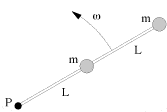# Rotational Inertia of a combination around a fixed axis

## Homework Statement

Two particles, each with mass m=0.0036kg, are fastened to each other and to a rotation axis at P, by two thin rods, each with length L=0.68m and each with a mass of 0.0086kg. The combination rotates around the rotation axis with an angular velocity of 13.0rad/s. Find the moment of inertia of the combination about P.## Homework Equations

I=Icm+Md2
Icm for thin rod about the end =1/3*M*L2
I=∫(x2+y2 dm and Rod is thin so y≈0 which makes
I=∫x2 dm and dm=M/L dx. After integration, I=1/3*ML2.

## The Attempt at a Solution

At first I just tried adding the lengths and masses and put that into I=1/3*ML2 but that didn't work. Then I tried using the center of mass = L/2, after some integration, and using that as the distance, d, in I=Icm+Md2 and that didn't work either. I don't know if I am supposed to multiply that number by two since there are two rod/particle combos or if the other one has a different center of mass or what. And I have no clue how or where to use the angular velocity unless it's in the conservation of mechanical energy equation. Any suggestions?

Well to start, are you sure the center of mass = L/2? Seems to me if you're working relative to P, that it would be 3L/2 .

$$I = m_1r_1^2 + m_2r_2^2 + m_3r_3^2 + m_4r_4^2 + ... = \sum_{i=1}^{n}m_ir_i^2$$

PS. The calculation of r_i is given with respect to the axis of rotation. In this case, P.

Last edited:
Can I find the r_i without knowing the radius or diameter of the particles....? Or is it simply 2L? I also tried using I=∫x2 dm with dm=M/2L dx which gave me M/2L * 8ML3/2L which is =4ML2. For total mass M, I did 2*0.0036kg+2*0.0086kg and just used L=0.68m. I ended up getting 0.04513kg*m2 and that was wrong too... What else can you give me?

To start, you should understand that the integral version for moment of inertia is for objects of continuous mass. Your system is very much a discrete mass system, which means you should stick to the summation.

You can think of the integral as some sort of limit of the summation for when the # of masses being considered goes to infinity. For example a disk has an infinite # of points, so it would be rather silly to try and apply the summation formula.

1) Generally in physics, a particle implies a "point particle". That is the particle has no radius or diameter, it is strictly a point.

2) Use the summation formula that defines moment of inertia and apply it to your system.
Hint:
$$I = m_1r_1^2 + m_2r_2^2$$

where, $$m_1 = m_2$$ and $$r_1$$ and $$r_2$$ are the distances from the axis of rotation (that is, the point P).

Doc Al
Mentor
At first I just tried adding the lengths and masses and put that into I=1/3*ML2 but that didn't work.
That's the rotational inertia of a rod, but it won't work for the two masses. What's the rotational inertia of a point mass at a distance R from an axis?

That's the rotational inertia of a rod, but it won't work for the two masses. What's the rotational inertia of a point mass at a distance R from an axis?

I=∫r2 dm???

Doc Al
Mentor
I=∫r2 dm???
Simpler than that (but on the right track). Note that this is a point mass, so the integration is trivial. (Reread Coto's posts; he gives the expression.)

Well I did
LaTeX Code: <BR>I = m_1r_1^2 + m_2r_2^2<BR>
using m1=m2=0.0244kg from 2*0.0086kg+2*0.0036kg. Then for r1=L and r2=2L. I ended up with I=0.0564kg*m2 and it was wrong. Do I need to use m1&2=0.0086+0.0036=0.0122kg?

Doc Al
Mentor
Well I did
LaTeX Code: <BR>I = m_1r_1^2 + m_2r_2^2<BR>
That would give you the rotational inertia of the two masses. (Don't forget the rods.)
using m1=m2=0.0244kg from 2*0.0086kg+2*0.0036kg.
The masses are given as m=0.0036kg. (Don't try to treat rods and masses as one, if that's what you're doing here. Find the rotational inertia of the masses and the rods separately, then add to get the total for the system.)
Then for r1=L and r2=2L.
OK.
I ended up with I=0.0564kg*m2 and it was wrong.
Fix the above errors. And you need the total I for the masses and the rods.

So it will be:
I=mparticle*L2+mparticle*2L2+mrod*L2+mrod*2L2?

=0.0282kg*m^2?

well that didn't work and now i'm ****ed.

Doc Al
Mentor
So it will be:
I=mparticle*L2+mparticle*2L2+mrod*L2+mrod*2L2?
No.

First term is correct; 2nd term is not (the distance is 2L). mR^2 works for a particle, not a rod. What's the rotational inertia of a rod?

The last two terms are incorrect. They are rods, not particles. Hint: If you connect two rods of length L together, what do you get?